# Prekinder to Grade 2 Mathematics

One way to tackle more difficult addition problems is to write one number being added below the other number, making sure you line up the ones, tens, and hundreds etc columns, and then add the two numbers, one column at a time, as in the examples below: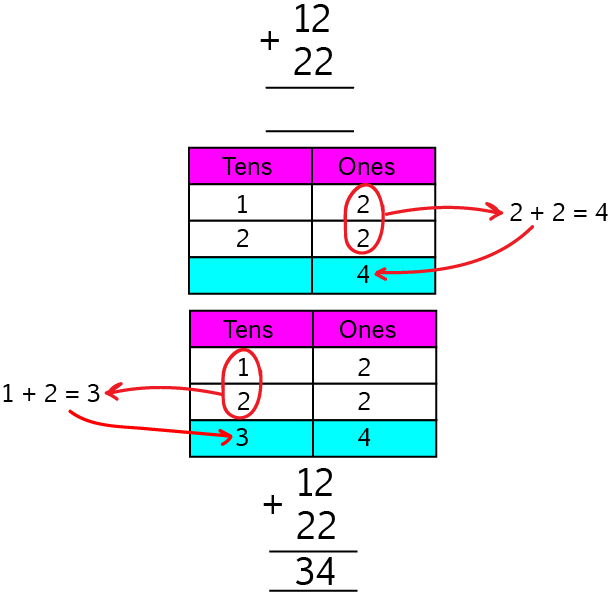It's always a good idea to add the ones column first, and then the tens column. Let's have a look at another example.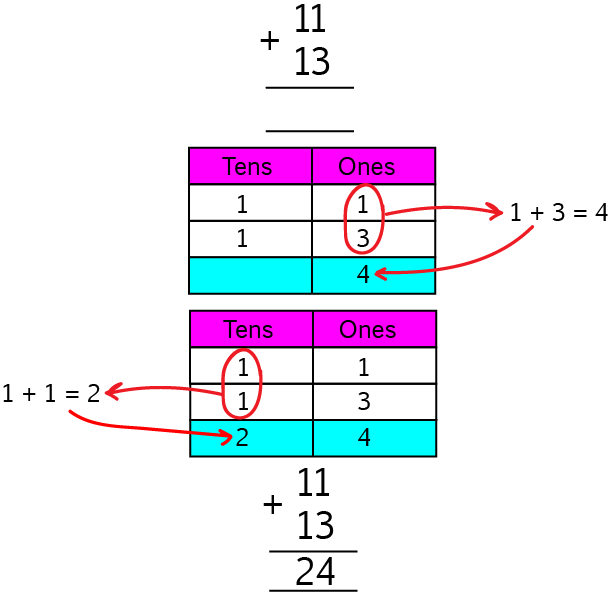When you add bigger numbers, the sum might spill over into the hundreds column like this: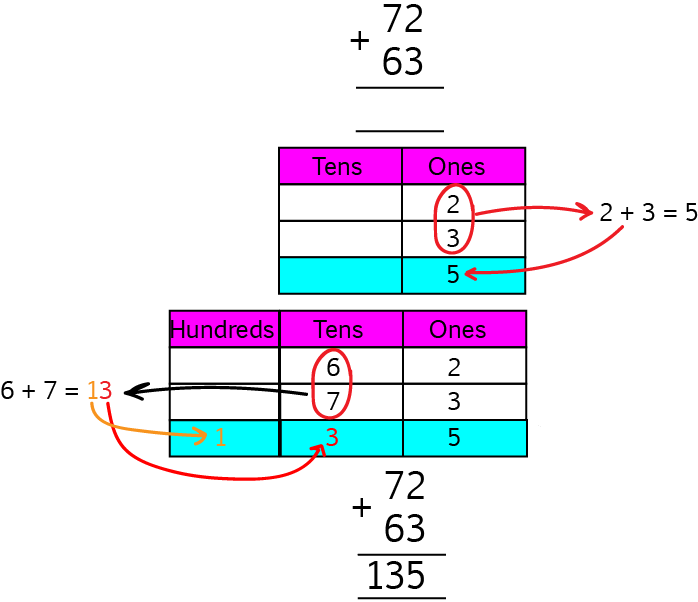Sometimes, when you add the numbers in the ones column you get a two digit number as the result. Then there isn't enough room to put it into the ones column. Have a look at what we do in this case in the example below: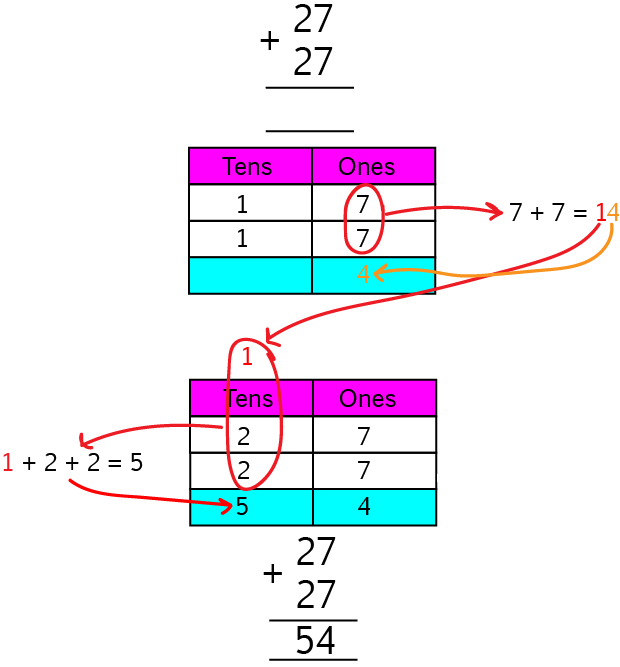Did you see what we did? We split the total of the ones column,$14$ up into its ones column, $4$, and its tens column, $1$. We then put the $1$ from the tens column of $14$ at the top of the tens column for the whole sum, and added it to the other two numbers in the tens column.

Let's have a look at another example: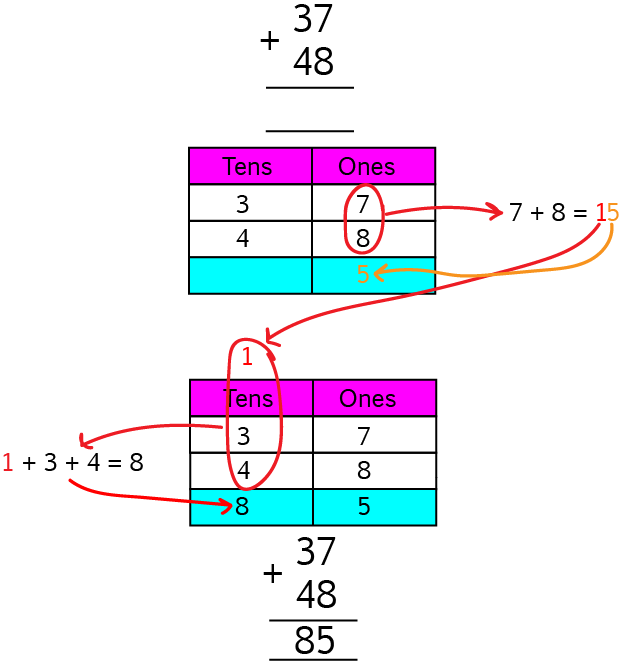When we added up the numbers in the ones column, we got $15$. We put the $5$ in the ones column of our answer and the $1$ up the top of the tens column of the sum. We then added the three numbers in the tens column of the sum to give the tens column for the answer.

You can also use this method to add three, four and five digit numbers, or any number of digits you like. Just remember to add one column at a time, and it's best to add the ones first, and move to the left.

Let's have a look at a few examples. We'll start with one where none of the columns add up to more than ten: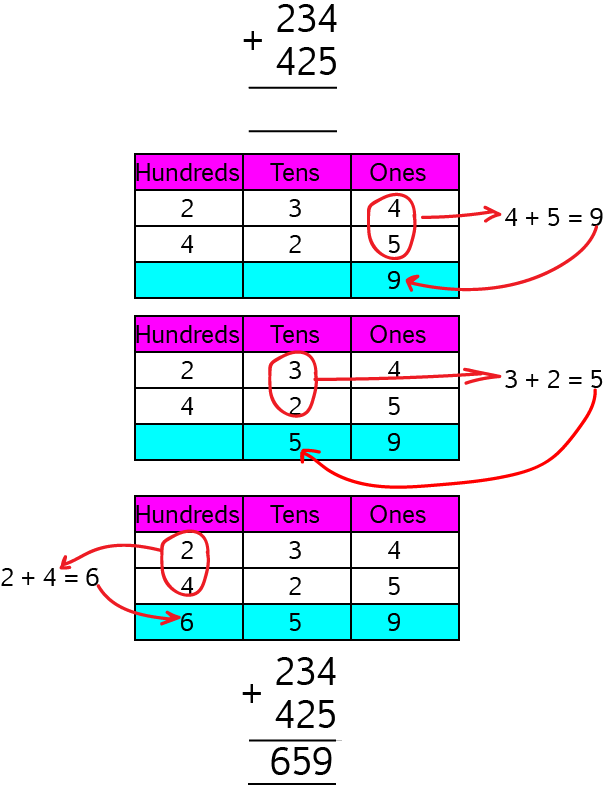See how we added the ones column, then the tens column, and finally the hundreds column? In the next example, the ones column adds to more than ten. We just use the same method we did for two-digit examples. You can use the same technique for any column that adds to more than ten. In really tricky examples, all of the columns will add to more than ten.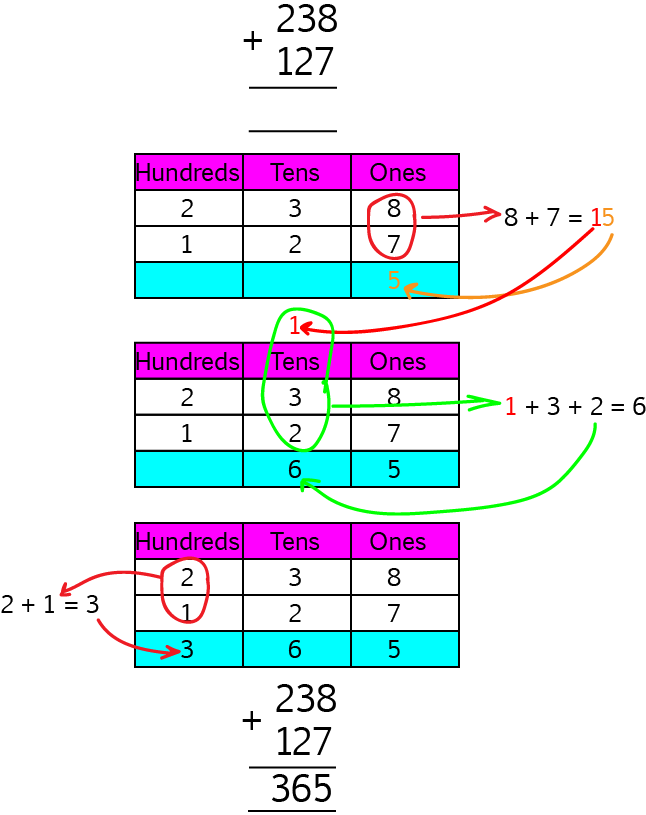The best thing you can do now is get lots of practice. Start with the simplest examples first: two digit numbers where no column adds to more than ten, and work your way up from there.

### Description

This mini book covers the core of Math for Foundation, Grade 1 and Grade 2 mathematics including

1. Numbers
3. Subtraction
4. Division
5. Algebra
6. Geometry
7. Data
8. Estimation
9. Probability/Chance
10. Measurement
11. Time
12. Money
13. and much more

This material is provided free of cost for Parent looking for some tricks for their Prekinder, Kinder, Prep, Year 1 and Year 2 children

### Learning Objectives

These lessons are for kids aged 4-8 with the core objective to expose their brains to concepts of addition, subtraction, division, algebra and much more.

Author: Subject Coach
You must be logged in as Student to ask a Question.